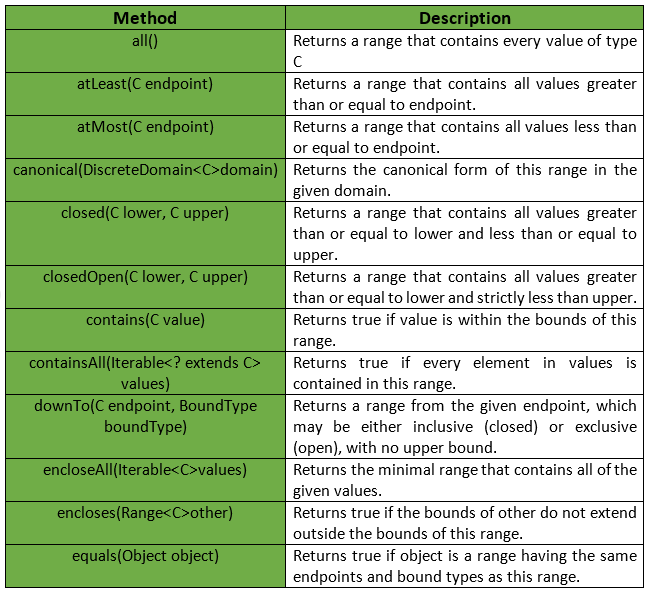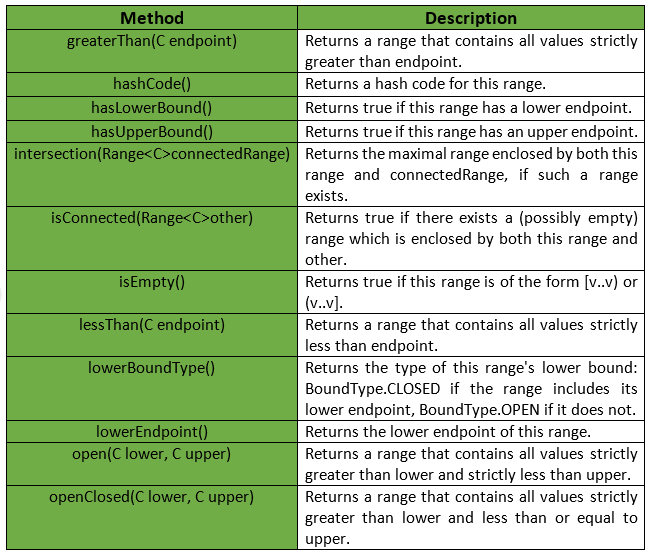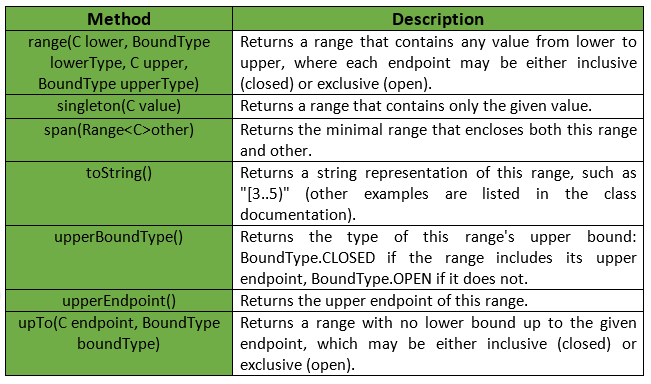GeeksforGeeks App
Open AppBrowser
Continue

# Range Class | Guava | Java

Guava’s Range represents an interval, for example, a < range < b. Here range includes any value between a and b, called endpoints which form the boundary. Any value between the boundary is a contiguous span of values of type Comparable.

Declaration : The declaration for com.google.common.collect.Range<C> class is :

```@GwtCompatible
public final class Range<C extends Comparable>
extends Object
implements Predicate<C>, Serializable
```

Each end of the range may be bounded or unbounded. If bounded, there is an associated endpoint value. If not it will be treated as infinity. A range can be further defined as either open or closed based whether the range is exclusive or inclusive of the endpoints.

• open(a, b) : It represents a < range < b, and in notation form, (a, b).
• closed(a, b) : It represents a <= range <= b, and in notation form, [a, b].
• openClosed(a, b) : It represents a < range <= b, and in notation form, (a, b].
• closedOpen(a, b) : It represents a <= range < b, and in notation form, [a, b).
• greaterThan(a) : It represents range > a, and in notation form, (a..+inf).
• atLeast(a, b) : It represents range >= a, and in notation form, [a..+inf).
• lessThan(a, b) : It represents range < b, and in notation form, (-inf..b).
• atMost(a, b) : It represents range <= b, and in notation form, (-inf..b].
• all() : It represents -inf < range < +inf, and in notation form, (-inf..+inf).

Below given are some methods provided by Range Class of Guava :Note : When both endpoints exist, the upper endpoint may not be less than the lower. The endpoints may be equal only if at least one of the bounds is closed :

• [a..a] : a singleton range.
• [a..a) or (a..a] : empty ranges, also valid.
• (a..a) : invalid, an exception will be thrown.

Some other methods provided by Range Class of Guava are :Exceptions :

• open : IllegalArgumentException if lower is greater than or equal to upper.
• closed : IllegalArgumentException if lower is greater than upper.
• closedOpen: IllegalArgumentException if lower is greater than upper.
• openClosed : IllegalArgumentException if lower is greater than upper.
• range : IllegalArgumentException if lower is greater than upper.
• encloseAll : ClassCastException if the parameters are not mutually comparable, NoSuchElementException if values is empty, NullPointerException if any of values is null.
• lowerEndpoint : IllegalStateException if this range is unbounded below (that is, hasLowerBound() returns false).
• lowerBoundType : IllegalStateException if this range is unbounded below (that is, hasLowerBound() returns false).
• upperEndpoint : IllegalStateException if this range is unbounded above (that is, hasUpperBound() returns false).
• upperBoundType : IllegalStateException if this range is unbounded above (that is, hasUpperBound() returns false).
• intersection : IllegalArgumentException if isConnected(connectedRange) is false.Below given are some examples to understand the implementation in a better way :
Example 1 :

 `// Java code to show implementation``// of Range class of Guava``import` `com.google.common.collect.Range;`` ` `class` `GFG {`` ` `    ``// Driver code``    ``public` `static` `void` `main(String[] args)``    ``{`` ` `        ``// Taking range (1, 5)``        ``// Note that here 1 and 5 are not included``        ``Range range = Range.open(``1``, ``5``);`` ` `        ``// Checking if range contains 1 or not``        ``System.out.println(range.contains(``1``));`` ` `        ``// Checking if range contains 2 or not``        ``System.out.println(range.contains(``2``));`` ` `        ``// Checking if range contains 3 or not``        ``System.out.println(range.contains(``3``));`` ` `        ``// Checking if range contains 4 or not``        ``System.out.println(range.contains(``4``));``    ``}``}`

Output :

```false
true
true
true
```

Example 2 :

 `// Java code to show implementation``// of Range class of Guava``import` `com.google.common.collect.Range;`` ` `class` `GFG {`` ` `    ``// Driver code``    ``public` `static` `void` `main(String[] args)``    ``{`` ` `        ``// Taking range [1, 5]``        ``// Note that here 1 and 5 are included``        ``Range range = Range.closed(``1``, ``5``);`` ` `        ``// Checking if range contains 1 or not``        ``System.out.println(range.contains(``1``));`` ` `        ``// Checking if range contains 2 or not``        ``System.out.println(range.contains(``2``));`` ` `        ``// Checking if range contains 3 or not``        ``System.out.println(range.contains(``3``));`` ` `        ``// Checking if range contains 5 or not``        ``System.out.println(range.contains(``5``));``    ``}``}`

Output :

```true
true
true
true
```

Example 3 :

 `// Java code to show implementation``// of Range class of Guava``import` `com.google.common.collect.Range;`` ` `class` `GFG {`` ` `    ``// Driver code``    ``public` `static` `void` `main(String[] args)``    ``{`` ` `        ``// Taking range (2, +inf)``        ``// Note that numbers less than equal to 2``        ``// are not included``        ``Range range = Range.greaterThan(``2``);`` ` `        ``// Checking if range contains 1 or not``        ``System.out.println(range.contains(``1``));`` ` `        ``// Checking if range contains 2 or not``        ``System.out.println(range.contains(``2``));`` ` `        ``// Checking if range contains 130 or not``        ``System.out.println(range.contains(``130``));`` ` `        ``// Checking if range contains 500 or not``        ``System.out.println(range.contains(``500``));``    ``}``}`

Output :

```false
false
true
true
```

Example 4 :

 `// Java code to show implementation``// of Range class of Guava``import` `com.google.common.collect.Range;`` ` `class` `GFG {`` ` `    ``// Driver code``    ``public` `static` `void` `main(String[] args)``    ``{`` ` `        ``// Taking range (-inf, 2]``        ``// Note that only numbers less than equal to 2``        ``// are included``        ``Range range = Range.atMost(``2``);`` ` `        ``// Checking if range contains 1 or not``        ``System.out.println(range.contains(``1``));`` ` `        ``// Checking if range contains 2 or not``        ``System.out.println(range.contains(``2``));`` ` `        ``// Checking if range contains -1 or not``        ``System.out.println(range.contains(-``1``));`` ` `        ``// Checking if range contains 5 or not``        ``System.out.println(range.contains(``5``));``    ``}``}`

Output :

```true
true
true
false
```

My Personal Notes arrow_drop_up
Related Tutorials